# JP3585701B2 - Cellular mobile communication system radio base station - Google Patents

## Info

Publication number
JP3585701B2
JP3585701B2 JP15502297A JP15502297A JP3585701B2 JP 3585701 B2 JP3585701 B2 JP 3585701B2 JP 15502297 A JP15502297 A JP 15502297A JP 15502297 A JP15502297 A JP 15502297A JP 3585701 B2 JP3585701 B2 JP 3585701B2
Authority
JP
Japan
Prior art keywords
signal
phase
output
antenna element
Prior art date
Legal status (The legal status is an assumption and is not a legal conclusion. Google has not performed a legal analysis and makes no representation as to the accuracy of the status listed.)
Expired - Fee Related
Application number
JP15502297A
Other languages
Japanese (ja)
Other versions
JPH114191A (en
Inventor

Original Assignee

Priority date (The priority date is an assumption and is not a legal conclusion. Google has not performed a legal analysis and makes no representation as to the accuracy of the date listed.)
Filing date
Publication date
Application filed by 富士通株式会社 filed Critical 富士通株式会社
Priority to JP15502297A priority Critical patent/JP3585701B2/en
Publication of JPH114191A publication Critical patent/JPH114191A/en
Application granted granted Critical
Publication of JP3585701B2 publication Critical patent/JP3585701B2/en
Anticipated expiration legal-status Critical
Application status is Expired - Fee Related legal-status Critical

## Images

••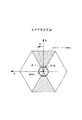•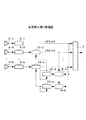••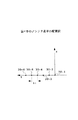•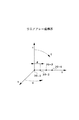•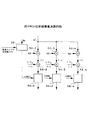•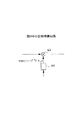•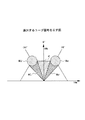•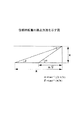•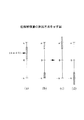•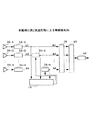•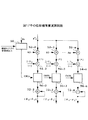•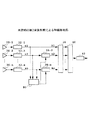•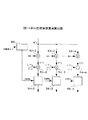•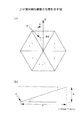••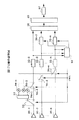•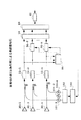•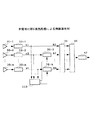•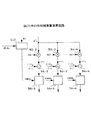•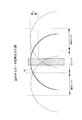## Classifications

• HELECTRICITY
• H01BASIC ELECTRIC ELEMENTS
• H01Q3/00Arrangements for changing or varying the orientation or the shape of the directional pattern of the waves radiated from an antenna or antenna system
• H01Q3/26Arrangements for changing or varying the orientation or the shape of the directional pattern of the waves radiated from an antenna or antenna system varying the relative phase or relative amplitude of energisation between two or more active radiating elements; varying the distribution of energy across a radiating aperture
• H01Q3/2605Array of radiating elements provided with a feedback control over the element weights, e.g. adaptive arrays
• HELECTRICITY
• H01BASIC ELECTRIC ELEMENTS
• H01Q3/00Arrangements for changing or varying the orientation or the shape of the directional pattern of the waves radiated from an antenna or antenna system
• H01Q3/26Arrangements for changing or varying the orientation or the shape of the directional pattern of the waves radiated from an antenna or antenna system varying the relative phase or relative amplitude of energisation between two or more active radiating elements; varying the distribution of energy across a radiating aperture
• H01Q3/267Phased-array testing or checking devices

## Description


TECHNICAL FIELD OF THE INVENTION
The present invention relates to a radio base station (hereinafter abbreviated as a radio base station) in a cellular mobile communication system, and more particularly, to phase compensation for correcting a phase shift when a received signal received by an antenna element directed to each sector is frequency-converted. Things.

[Prior art]
Various mobile communication services such as automobile and mobile phone services have been remarkably developed in recent years not only in Japan but also worldwide. Among mobile communications, the development of cellular / mobile phone services by the cellular system is particularly remarkable.

A cellular mobile communication system is a mobile communication system that covers a service area with a large number of cells that are service zones of each radio base station. Each cell is divided into a plurality of, for example, six sectors in order to reduce interference with cells in directions other than the directivity direction of the antenna element and to remove interference waves arriving from mobile stations in directions other than the directivity direction. Then, a plurality of antenna elements pointing to each sector receive signals from mobile stations located in the sector.

Received signals received by a plurality of antenna elements directed to each sector are low-noise amplified by an LNA (Low Noise Amplifier), and received by a heterodyne receiving unit including an AGC circuit. A Q signal is generated (hereinafter, a circuit formed of non-linear elements until a received signal is converted to a baseband is called a receiver), and the beamformer gives an amplitude weight and a phase rotation, and synthesizes the desired signal. To improve the gain.

Applying digital signal processing multi-beam antennas and adaptive array antennas to the radio base station of a cellular mobile communication system can improve the gain by sharpening the beam pattern and reduce the interference in the area. Thus, the number of users that can be accommodated in one cell can be relatively easily increased.

[Problems to be solved by the invention]
However, the conventional beam shaping by digital signal processing is still in the research stage for the radio base station of the cellular mobile communication system, and the ideal environment (the output of the receiver of the reception signal of each antenna element (hereinafter referred to as antenna branch) Most computer simulations assume that there is no phase deviation between the two.

In the case of multi-beam antenna molding, in order to form a beam of the output of a plurality of antenna branches assuming that there is no phase deviation between antenna branches, nonlinear elements such as LNAs and mixers, manufacturing errors, aging, and temperature characteristics For example, when there is a phase deviation between antenna branches due to the above-described problem, a desired beam pattern cannot be obtained, and there is a problem that characteristics are deteriorated.

In addition, in the case of adaptive array antenna shaping, even if there is a phase deviation between each antenna branch, it is conceivable that there is no problem because the amplitude and phase are controlled, including the phase deviation. Separating the control amount of the phase is a necessary condition when performing transmission beam shaping from the control amount at the time of transmission at the time of transmission. However, conventionally, this control amount could not be separated.

The present invention has been made in view of such a point, and uses a specific user signal in a sector to compensate for a phase deviation due to a non-linear element of a receiver in a stage before beam shaping in a digital domain, thereby improving efficiency. An object of the present invention is to provide a cellular mobile communication system radio base station that performs good beamforming.

[Means for Solving the Problems]
FIG. 1 is a first principle diagram of the present invention.
FIG. 2 is a diagram showing a sector.

In FIG. 2, the radio base station is located at the center and controls mobile stations located within a certain range of cells. The cell is divided into a plurality of (for example, six) sectors, and antenna elements 2-k (k = 1 to n) pointing to each sector are arranged.

Since the same can be said for any of the sectors, the sectors indicated by oblique lines in FIG. 2 will be described. A plurality of antenna elements 2-k (k = 1 to n) having directivity in this sector are arranged parallel to the Y axis.


Since the invention is configured as described above, assuming that the number of users in the area of this sector is m and the number of antenna elements 2-k (k = 1 to n) directed to the sector is n, the receiver 4- The k (k = 1 to n) user signals Xk (k = 1 to n) are represented by complex numbers as in the following equation (1).

X1 = exp [(-1)1/2i(T) + φ1)]
X2 = exp [(-1)1/2i(T) + Δθi2+ Φ2)]

Xn = exp [(-1)1/2i(T) + Δθin+ Φn)] ・ ・ ・ (1)
However,
αi(T): phase of each reception path of the ith (i = 1 to k) th user signal
Δθij: The phase rotation of Xj determined by the arrangement of the antenna element 2-k and the angle of arrival of the i-th user with reference to X1
φj: Phase including phase deviation of receiver 4-j
(-1)1/2: Imaginary unit
Using this signal,
Yj = Xj · X*1 = exp [(-1)1/2j−φ1+ Δθij)] ・ ・ ・ (2)
Is calculated.

However,
*: Complex conjugate
The phase compensation amount calculation means 8-j receives the output signals X1 and Xj of the first and second receivers 4-1 and 4-j and receives the phase compensation amount ΔΦ = (φj−φ1  ) Is calculated.

The phase compensator 10-j performs the phase compensation of the following equation (3) on the output signal Xj of the second receiver 4-j.
Aj = Xj.exp (-(-1)1/2ΔΦ) = exp [(− 1)1/2i(T) + φ1+ Δθij)] ... (3)
by the way,
X1 = A1 = exp [(-1)1/2i(T) + φ1)] ・ ・ ・ (4)
Therefore, the phase term of the output signal Aj of the phase compensating means 10-j is a phase rotation term Δθ determined by the antenna arrangement and the angle of arrival of each user.ijExcept for, the receiving path is the same as the reference receiving path of the antenna element 2-1, and phase compensation is performed.

The beamformer 6 performs beamforming into a desired beam pattern based on the phase-compensated signal. Since the signal input to the beam former 6 is phase-compensated by the phase compensating means 10-j, the efficiency of beam pattern shaping is improved in the case of multi-beam antenna shaping.

Further, the phase compensation amount calculating means 8-k outputs the first antenna element 2-2 in the uplink signal from the mobile station located in the sector to which the first and second antenna elements 2-1, 2 -j are directed. 1 and the second receiver 4-1 of the upstream signal belonging to a population whose average value of the phase rotation amount indicating the phase difference between the output signal of the second antenna element 2-j and the output signal of the second antenna element 2-j is a known value. , 4-j that outputs a control signal indicating that the output is the output of the first receiver 2-1 and the average of the phase difference between the output signal of the first receiver 2-1 and the second receiver 2-j based on the control signal. An averaging means for calculating a difference between the value and the known value as a phase compensation amount may be provided.

As shown in Expression (2), the phase difference between the output signal X1 of the first receiver 4-1 and the output signal Xj of the second receiver 4-j is (φj−φ1+ Δθij). The control means is ΔθijIs the known value ΔΘjThe averaging means is controlled so as to use an upstream signal belonging to a population such that

The averaging means calculates an average value Bj of the phase difference between X1 and Xj expressed by the following equation (5).

(Equation 1)

Bj shown in equation (5) is (φj−φ1+ ΔΘj). Then, the amount of phase compensation is ΔΘ from Bj.jIs subtracted, the output becomes (φj−φ1). The phase of Xj (αi(T) + Δθij+ Φj) To (φj−φ1) To correct the phase. Thus, the output of the phase compensating means 10-j is phase-compensated as shown in Expression (3).

FIG. 3 is a second principle diagram of the present invention.
According to the present invention, as shown in FIG. 3, a phase compensation means for inputting a first phase compensation amount and correcting a phase of an output signal of the second receiver 4-j based on the first phase compensation amount. 12-j, the output signal of the first receiver 4-1 and the output signal of the phase compensator 10-j are input, and the output signal of the first receiver 4-1 and the output signal of the phase compensator 10-j Compensation amount calculating means 8-j for calculating a second phase compensation amount indicating a phase difference between the phase difference between the first and second input signals of the first and second receivers 4-1 and 4-j. And an adder 12-j that adds the second phase compensation amount and its own output signal and outputs the first phase compensation amount to the phase compensator 10-j.

The second phase compensation amount indicates a phase compensation amount that must be phase compensated for the output of the phase compensating means 10-j. By adding its own output, which is the current phase compensation amount, to the second phase compensation amount, which is the output from the phase compensation amount calculating means 8-j, by the adding means 12-j, the overall output for the second receiver 4-j is obtained. The first phase compensation amount is obtained.

Then, the phase compensator 8-j performs phase compensation on the output of the second receiver 4-j based on the first phase compensation amount. As a result, the output of the phase compensator 10-j becomes equal to the equation (3), and a signal having the same phase is input to the beam former 6. In the case of the multi-beam antenna molding, the beam pattern The efficiency of molding is improved.

In addition, the phase compensation amount calculating means 8-j performs the second compensation for the output signal of the first antenna element 2-1 in the uplink signal from the mobile station located in the sector to which the first and second antenna elements are directed. This is the output of the first receiver 4-1 and the phase compensator 10-j of the upstream signal belonging to the population whose average value of the phase rotation amount indicating the phase difference of the output signal of the antenna element 2-j is a known value. Control means for outputting a control signal indicating that the average value and the known value of the phase difference between the output signal of the phase compensation means 10-j and the output signal of the first receiver 4-1 are based on the control signal. An averaging means for calculating the difference as the phase compensation amount may be provided.

Further, the control means performs inter-sector handover to a mobile station located near the boundary between the sector to which the first and second antenna elements 2-1 and 2-j point and a specific adjacent sector of the sector. Averaging means generates a control signal indicating that the output signal is based on the uplink signal, and calculates, as a known value, an average position of the mobile station to be subjected to the inter-sector handover and the first and second antenna elements. May be used.

Generally, the amount of phase rotation of the output of the second antenna element 2-j with respect to the output of the first antenna element 2-1 is equal to the uplink rotation with respect to the arrangement direction of the antenna elements 2-1 and 2-j, assuming that the uplink signal is a plane wave. It is determined by the angle of arrival of the signal.

Therefore, from the average position of the population of mobile stations and the positions of the antenna elements 2-1, 2-j, the known value ΔΘjIs obtained in advance. This known value ΔΘjIs used to calculate the amount of phase compensation to perform phase compensation.

Further, the control means outputs an output signal based on an uplink signal performing an inter-cell handover to a mobile station located within a certain range from a specific center located at a certain distance from the cellular mobile communication system radio base station. And the averaging means uses, as a known value, a value obtained in advance based on the position of the specific center and the positions of the first and second antenna elements. You may.

Further, the control means controls an output signal of an arbitrary third antenna element directed to a specific adjacent sector of the first sector directed by the first and second antenna elements 2-1 and 2-j, and directs the output signal of the first sector. A control signal indicating that the output signal is an output signal based on an uplink signal in which the level difference of the output signal of any fourth antenna element is equal to or smaller than a threshold, and the averaging means determines that the level difference is a known value. An average position of the mobile station that is equal to or less than a threshold, a position of the first and second antenna elements, and a value obtained in advance based on a radiation pattern of the third and fourth antenna elements may be used.

Since the third and fourth antenna elements each form a unique radiation pattern, the difference between the level of the output signal of any antenna element pointing to the adjacent sector and the level of the output signal of any antenna element pointing to the first sector is a threshold. The following are presumed to be uplink signals from near the boundary with the adjacent sector.

Therefore, the range near the boundary is obtained in advance by calculation or the like, and the phase rotation amount ΔΘ is calculated from the average position and the positions of the first and second antenna elements 2-1 and 2-j.jCan be estimated. This phase rotation amount ΔΘjCan be used to calculate the amount of phase compensation.

Further, the control means may control the output signals of arbitrary third and fourth antenna elements pointing to two adjacent sectors adjacent to the first sector to which the first and second antenna elements 2-1 and 2-j point, respectively. A control signal is generated which indicates that the output signal is based on an uplink signal whose level difference from an output signal of an arbitrary fifth antenna element directed to the first sector is equal to or greater than a threshold value, and the averaging means generates a control signal. , The average position of the mobile station where the level difference is equal to or greater than the threshold value, the positions of the first and second antenna elements 2-1, 2-j, and the third, fourth, and fifth antenna elements. May be used in advance based on the radiation pattern.

Since the uplink signal whose level difference is equal to or larger than the threshold value is estimated to be located near the center of the sector, the average position and the first and second antenna elements 2-1 and 2-1 near this sector are located near the sector. −j from the position of phase rotation ΔΘjCan be estimated. This phase rotation amount ΔΘjCan be used to calculate the amount of phase compensation.

Further, the apparatus further comprises a signal generator for transmitting a signal from a known position in a sector to which the first and second antenna elements are directed. Based on the phase difference between the output signal of the first antenna element 2-1 and the output signal of the second antenna element 2-j obtained from the positions of the first and second antenna elements, an upstream signal from the signal generator is used. Alternatively, the phase compensation amount or the second phase compensation amount may be calculated.

In addition, the apparatus further includes a signal generator for transmitting a signal from a known position in a sector to which the first and second antenna elements 2-1 and 2-j are directed. And the phase difference between the output signal of the first antenna element 2-1 and the output signal of the second antenna element 2-j obtained from the positions of the first and second antenna elements 2-1 and 2-j. , A phase compensation amount or a first phase compensation amount may be calculated using output signals of the first and second receivers of the signal from the signal generator.

Also, a signal generator that generates a signal in the same band as the wireless band used by the cellular mobile communication system and outputs the signal to the signal line, and a first signal based on the signal from the signal line to the first receiver 4-1. First output means for outputting the first signal, and second output means for outputting a second signal based on the signal from the signal line to the second receiver 4-j. Based on a known phase difference with the second signal, the phase compensation amount is calculated using an output signal of the first receiver 4-1 of the first signal and an output signal of the second receiver 4-j of the second signal. Alternatively, the first phase compensation amount may be calculated.

Further, the apparatus further comprises switch means for outputting either the first signal based on the output signal of the first antenna element 2-1 or the output signal of the second antenna element 2-j to the second receiver 4-j, The compensation calculating means is configured to determine the output signal of the first receiver 4-1 and the second receiver 4-j of the first signal based on a known phase difference between the output signal of the first antenna element 2-1 and the first signal. May be used to calculate the phase compensation amount or the first phase compensation amount.

BEST MODE FOR CARRYING OUT THE INVENTION
Hereinafter, embodiments of the present invention will be described with reference to the drawings.
First embodiment
FIG. 4 is a block diagram of the radio base station according to the first embodiment of the present invention.

As shown in the figure, the radio base station is, for example, a cellular mobile communication system of the TDMA scheme, and a plurality of antenna elements 30-k (k = 1 to n) pointing to the same sector, and a plurality of antenna elements 30-k. For each branch, a receiver 32-k (k = 1 to n), a phase compensation amount calculation circuit 34, a phase compensation circuit 36-j (j = 2 to n), a beamformer 38, a beam selector 40, and a demodulation circuit 42 Have.

FIG. 5 is an arrangement diagram of the antenna elements in FIG.
FIG. 6 is a diagram showing a linear array coordinate system.
As shown in FIGS. 5 and 6, when the center direction of a sector is the X axis and the axis orthogonal to the X axis in the horizontal plane is the Y axis, the antenna element 30-k (k = 1 to n) They are arranged in the Y-axis direction for pointing. The Z axis in FIG. 6 is taken in a direction orthogonal to the X axis and the Y axis so as to form a right-handed system.

In the linear array system, the antenna elements 30-k (k = 1 to n) are usually arranged at equal intervals d (for example, equal to the wavelength λ of the uplink received signal). Hereinafter, description will be made with reference to the antenna element 30-1 arranged at the origin of the linear array coordinate system. The angle ψ between a straight line connecting the origin and the mobile station and the X axis, and the angle の between a straight line connecting the origin and the mobile station and the Z axis ξ.

The receiver 32-k (k = 1 to n) extracts a predetermined frequency component from the output signal of the antenna element 30-k and generates digital orthogonal baseband signals Ik and Qk. Although not described, it is composed of an LNA and a heterodyne receiving unit including an AGC circuit.

FIG. 7 is a configuration diagram of the phase compensation amount calculation circuit in FIG.
As shown in this diagram, the phase compensation amount calculation circuit 34 includes a control circuit 50, a multiplier 52-j (j = 2 to n), an adder 54-j (j = 2 to n), and an averaging unit 56-. j (j = 2 to n).

The control circuit 50 is a circuit that generates a control signal CNTL for selecting a target for averaging the output signal of the adder 54-j in the averaging unit 56-j (j = 2 to n). , The circuit is configured according to the target for taking the average value.

For example, when selecting a user signal performing a handover between sectors with a specific adjacent sector as a target, the control circuit 50 determines, for example, the power of the output signal Xk of one receiver 32-k and one of the adjacent sectors. This is a circuit configuration for generating a control signal CNTL indicating that the power of the output signal of one receiver has reached the level at which handover between sectors is performed.

The multiplier 52-j (j = 2 to n) outputs the complex conjugate X of the output signal X1 of the receiver 32-1.*1 (= (I1-(-1)1/2Q1)) and the output signal Xj (= (Ij + (-1)) of the receiver 32-j.1/2Qj)).

The adder 54-j calculates the phase argYj of the output Yj of the multiplier 52-j, and from the average position of the mobile station whose average value is to be calculated by the averaging unit 56-j based on the phase argYj, as described below. Mean value ΔΘ of the phase rotation amount of the reception signal of antenna element 30-j with respect to the reception signal of antenna element 30-1 calculated in advance.jIs subtracted. The position of the adder 54-j may be after the averaging unit 56-j.

In a coordinate system such as that shown in FIG.i, Angle from Z axis 軸iIs received by each antenna element 30-j (j = 2 to n) from the i-th user located in the antenna signal, the uplink signal is a plane wave, the antenna element 30-1 is the origin, and the origin of the antenna element 30-j is Assuming that the distance from the antenna element 30-1 is d (j-1), the phase rotation amount ?? of the reception signal of the antenna element 30-j with respect to the reception signal of the antenna element 30-1 of the i-th user signal.ijIs as shown in the following equation (6).

Δθij= (2π / λ) (j-1) dsinψi・ Sinξi    ... (6)
However
λ: wavelength of uplink signal of mobile station
The average value ΔΘj(J = 2 to n) is the phase rotation amount Δθ for a plurality of target users.ijThis is the average value of the user, and specifies the range of the target for which the average value is to be determined, and substitutes the average position of the users in the target range into Expression (6) to obtain the average in advance.

Although not shown, the averaging unit 56-j (j = 2 to n) includes a delay circuit and an averaging circuit. The delay circuit is for synchronizing the control signal CNTL of the control circuit 50 with the output of the adder 54-j. The adder 54-j delays the control signal CNTL with respect to the output of the adder 54-j. Is to delay the output. The averaging circuit obtains a temporal average value of the output of the delay circuit when the control signal CNTL indicates a selection instruction (hereinafter, referred to as active).

FIG. 8 is a diagram showing the phase compensation circuit in FIG.
The phase compensation circuit 36-j applies the phase correction amount Δφ calculated by the phase compensation amount operation circuit 34 to the output Xj of the receiver 32-j.jThe phase is corrected only by the phase correction amount Δφ as shown in FIG.jFrom exp [-(-1)1/2ΔφjAnd an arithmetic circuit 60 for calculating Xj.exp [-(-1)1/2Δφj] Is obtained.

The beamformer 38 outputs an arbitrary amplitude weight and phase rotation to the output X1 (A1) of the receiver 32-1 and the output Aj of the phase-compensated phase compensation circuit 36-j (j = 2 to n). And a digital signal processing circuit composed of a multi-beam antenna or an adaptive array antenna that forms a desired beam pattern by combining the signals.

The beam selector 40 selects a beam having the highest level of a desired user signal from a plurality of outputs of the beam former 38. The demodulation circuit 42 is a circuit that demodulates a modulation signal such as π / 4 shift QPSK.

Hereinafter, the operation of the wireless base station according to the first embodiment will be described with reference to these drawings.
For example, an uplink signal of a user pointing to a hatched sector in FIG. 2 is received by the antenna elements 30-k (k = 1 to n), and the received signal is sent to the receiver 32-k. The receiver 32-k converts the signal into orthogonal digital signals Ik and Qk by a low noise amplification by LNA, amplitude equalization by AGC, and a heterodyne receiver.

Here, the output Xk of the user signal from the receiver 32-k is represented by the following equation (7).
Xk = exp [(-1)1/2i(T) + (k-1) Δθi+ Φk)] ・ ・ ・ (7)
However,
αi(T): phase of each reception path of the i-th user signal
Δθi: A phase rotation determined by the antenna element interval d and the angle of arrival from the i-th user with reference to X1.

φk: Phase including phase deviation of receiver 32-k
Here, since the amplitude deviation is compensated by AGC, the amplitude is constant.
The output X1 of the receiver 32-1 is input to the beamformer 38, and the complex conjugate X of the output X1 is obtained.*1 is input to the phase compensation amount calculation circuit 34. The output Xj of the receiver 32-j (j = 2 to n) is input to the phase compensation amount calculation circuit 34 and the phase compensation circuit 36-j.

On the other hand, the control circuit 50 in FIG. 7 receives an output Xk of one receiver 32-k (i = 1 to n), an output of a receiver of a reception signal of one antenna element directed to an adjacent sector, and the like. Then, a control signal CNTL for selecting an uplink user signal in the following range is generated and output to the averaging section 56-j (j = 2 to n).

FIG. 9 is a diagram illustrating a user to be selected.
As shown in this figure, the user to select is
A user Hr who is performing a handover between the right adjacent sector and the sector
The user Hl performing a handover between the adjacent sector to the left and the sector
・ User Sr performing inter-cell handover in the upper right area
-User Sl performing inter-cell handover in the upper left area
-User C in the sector excluding the above-mentioned users Hr, Hl, Sr, Sl
It is.

(A) User Hr performing handover between sectors adjacent to the right and sectors
For example, the control circuit 50 controls the power of the output Xk of one receiver 32-k (for example, the power of the output obtained by detecting the output Xk) and the reception signal of one antenna element pointing to the right adjacent sector. When the power of the output Xr of the receiver reaches a level at which inter-sector handover is performed, the control signal CNTL is activated. At other times, the control signal CNTL is deactivated and the averaging unit 56-j (j = 2 to n).

In this case, the user Hr finds that, on average, a signal arrives from the boundary direction with the right adjacent sector. That is, the user signal arrives at ψ = 30 ° from a distance of 1 from the radio base station.

Therefore, Δθ shown in equation (6)ijAre averaged, it is estimated that ψ = 30 °, which is equal to the phase rotation amount of the user signal located at a distance of 1 from the radio base station. Therefore, ΔΘjCan be obtained by calculating the amount of phase rotation in advance.

FIG. 10 is a diagram illustrating a method of calculating the amount of phase rotation.
Since the radio base station manages an area having a radius R from the center as a cell, it is assumed that a user performing inter-sector handover is located at an average distance of l = R / √2 from the radio base station. Presumed.

As shown in FIG. 10, assuming that the height of the antenna element 30-k (k = 1 to n) of the wireless base station is H, the antenna element 30-k viewed from a user located at R / √2 from the wireless base station is shown. The elevation angle α of −k is expressed by the following equation (8).

α = tan-1(√2H / R) (8)
Therefore, in the coordinate system shown in FIG.i, Ξi) = (Π / 6, α + π / 2). By substituting this into equation (6), ΔΘjIs calculated. This ΔΘjAre input to the (-) terminal of the adder 54-j in FIG.

On the other hand, the multiplier 52-j outputs the complex conjugate X of the receiver 32-1.*1 is multiplied by the output Xj of the receiver 32-j, and the output Yj of the multiplier 52-j represented by the following equation (9) is output to the adder 54-j.

Yj = Xj · X*1 = exp [j (φj−φ1+ (J-1) Δθi)] ・ ・ (9)
The adder 54-j inputs the phase argYj (−π ≦ argYj ≦ π) of the output Yj of the multiplier 52-j to the (+) terminal, and (j−1) ΔθiAverage Δ 値jInto the (−) terminal, and argYj−ΔΘjIs calculated and output to the averaging unit 56-j.

The averaging unit 56-j receives the output of the adder 54-j, delays it by a delay circuit, and synchronizes with the control signal CNTL. If the control signal CNTL is active by the averaging circuit, a temporal average value is obtained for the output of the stacked adder 54-j, and the phase compensation circuit 36-j in FIG. Compensation amount ΔφjIs output.

The phase of equation (9) (φj−φ1+ (J-1) Δθi) Is (φj−φ1+ ΔΘj), The output Δφ of the averaging unit 56-jjIs (φj−φ1).

Here, -π ≦ argYj−ΔΘjSince ≦ π, as shown in FIG. 11A, {argYj−Δ}jThere is no problem if the set of｝ is continuous, but as shown in FIG. 4B, when the set is separated into a first part having an upper limit of π and a second part having a lower limit of −π, If the average value is obtained as it is, a correct result cannot be obtained. Therefore, the averaging unit 56-j shifts the second portion by 2π as shown in FIG. Thus, the first portion is shifted by -2π and adjusted so as to be continuous, and then the average value is obtained.

The phase compensating circuit 36-j is operated by the arithmetic unit 60 in FIG.j] Is output to the multiplier 62. The multiplier 62 adds exp [-jΔφ to the output Xj of the receiver 32-j.jAnd outputs Aj shown in the following equation (10) to the beamformer 38.

Aj = Xj.exp [-(-1)1/2Δφj] = Exp [(-1)1/2i(T) + φ1+ (J-1) Δθi)] ・ ・ ・ (10)
by the way,
X1 = A1 = exp [(-1)1/2i(T) + φ1)] ・ ・ ・ (11)
Therefore, the phase term of the j-th reception path is the same as the first reception path as a reference, except for the phase rotation term determined by the antenna arrangement and the angle of arrival of each user, and phase compensation is performed.

The beam former 38 receives the output A1 (= X1) of the receiver 31-1 and the output Aj (j = 2 to n) of which the phase is compensated, and outputs a digital signal by a multi-beam antenna or an adaptive array antenna system configuration. Perform processing. In the multi-beam antenna, since the input phase is compensated, the efficiency of beam shaping is improved.

The beam selector 40 selects a beam having the highest level of the desired user signal from the plurality of outputs of the beam former 38 and outputs the selected beam to the demodulation circuit 42. The demodulation circuit 42 demodulates a modulation signal such as π / 4 shift QPSK.

(B) User Hl performing handover between adjacent sectors to the left
On average, the user Hl knows that the signal is coming from the boundary direction with the left adjacent sector. That is, the user signal arrives at ψ = 30 ° from a distance of R / √2 from the radio base station. Therefore, in the coordinate system shown in FIG.i, Ξi) = (− Π / 6, α + π / 2). By substituting this into equation (6), the average value ΔΘjIs obtained.

This ΔΘjIn the same manner as in the case (a), the phase compensation amount calculation circuit 34 uses the phase compensation amount ΔφjAnd the phase compensation circuit 36-j calculates Xj · exp [-(− 1) for Xj.1/2Δφj] And outputs it to the beamformer 38.

(C) User Sr performing handover between cells in the upper right area
On average, the user Sr knows that the signal is coming from the direction located at the center. That is, the user signal arrives at ψ = 30 ° from a distance of R from the radio base station. Therefore, as shown in FIG. 10, the position coordinates (ψi, Ξi) = (Π / 6, β + π / 2) (β = tan-1(H / R)). By substituting this into equation (6), the average value ΔΘjIs obtained.

This ΔΘjIn the same manner as in the case (a), the phase compensation amount calculation circuit 34 uses the phase compensation amount ΔφjAnd the phase compensation circuit 36-j calculates Xj · exp [−j Δφ with respect to Xj.j] And outputs it to the beamformer 38.

(D) User Sl performing inter-cell handover in the upper left area
On average, the user Sl is estimated to have received a signal from the direction located at the center. That is, it is estimated that the user signal arrives at ψ = −30 ° from the distance of R from the radio base station. Therefore, as shown in FIG. 10, the position coordinates (ψi, Ξi) = (− Π / 6, β + π / 2) (β = tan-1(H / R)). By substituting this into equation (6), the average value ΔΘjIs obtained.

This ΔΘjIn the same manner as in the case (a), the phase compensation amount calculation circuit 34 uses the phase compensation amount ΔφjAnd the phase compensation circuit 36-j calculates Xj · exp [−j Δφ with respect to Xj.j] And outputs it to the beamformer 38.

(E) User C
On the average, the user C is estimated to have received a signal from the center of the sector. That is, it is estimated that the user signal arrives from ψ = 0 ° and a distance of R / √2 from the radio base station. Therefore, the user's position coordinates (ψi, Ξi) = (0, α + π / 2). By substituting this into equation (6), the average value ΔΘj= 0 is obtained.

This ΔΘj= 0, the phase compensation amount calculation circuit 34 performs the phase compensation amount Δφ in the same manner as in the case (a).jAnd the phase compensation circuit 36-j calculates Xj · exp [−j Δφ with respect to Xj.j] And outputs it to the beamformer 38.

According to the first embodiment described above, the phase compensation amount is calculated by the phase compensation amount calculation circuit 34, and the phase compensation is performed on the output Xj of the receiver 32-j by the phase compensation circuit 36-j. Therefore, a signal having the same phase is input to the beamformer 38, and the beamformer of the multi-beam antenna system improves the efficiency of beamforming. Further, in the case of the beamformer of the adaptive antenna system, the phase deviation, the amplitude of adaptive processing, and the control amount of the phase can be separated, and transmission beam shaping can be performed based on the phase deviation amount at the time of reception at the time of transmission.

Second embodiment
FIG. 12 is a block diagram of a radio base station according to the second embodiment of the present invention. Elements having the same functions as the elements in FIG. 4 are denoted by the same reference numerals.

As shown in this figure, the radio base station is, for example, a cellular mobile communication system of the TDMA system, and a plurality of antenna elements 30-k (k = 1 to n) pointing to the same sector, and a receiver 32-k ( k = 1 to n), a phase compensation amount calculation circuit 70, a phase compensation circuit 36-j (j = 2 to n), a beam former 38, a beam selector 40, and a demodulation circuit 42.

The antenna element 30-k, the receiver 32-k (k = 1 to n), the phase compensation circuit 36-j (j = 2 to n), the beam former 38, the beam selector 40, and the demodulation circuit 42 in FIG. And the description is omitted.

FIG. 13 is a configuration diagram of the phase compensation amount calculation circuit in FIG. 12, and elements having the same functions as the elements in FIG. 7 are denoted by the same reference numerals.
As shown in this figure, the phase compensation amount calculation circuit 70 includes a control circuit 50, a multiplier 52-j (j = 2 to n), an adder 54-j (j = 2 to n), and an averaging unit 56-. j (j = 2 to n) and an adder 72-j (j = 2 to n).

The description of the control circuit 50 that is the same as that in FIG. 7 is omitted. The multiplier 52-j (j = 2 to n) outputs the complex conjugate X of the output X1 of the receiver 32-1.*1 is multiplied by the output Aj of the phase compensation circuit 54-j. The adder 54-j and the averaging unit 56-j (j = 2 to n) are the same as those in FIG. The adder 72-j adds the output of the averaging section 56-j and its own output.

Hereinafter, the operation of the wireless base station according to the second embodiment will be described with reference to these drawings.
For example, an uplink signal of a user pointing to a hatched sector in FIG. 2 is received by the antenna elements 30-k (k = 1 to n), and the received signal is sent to the receiver 32-k. The receiver 32-k converts the signal into orthogonal digital signals Ik and Qk by a low noise amplification by LNA, amplitude equalization by AGC, and a heterodyne receiver.

The output X1 of the receiver 32-1 is input to the beamformer 38, and the complex conjugate X1 of X1 is output.*1 is input to the phase compensation amount calculation circuit 70. The output Xj of the receiver 32-j (j = 2 to n) is input to the phase compensation circuit 36-j.

The phase compensation circuit 36-j outputs the output Xj of the receiver 32-j and the phase amount Δρ output from the phase compensation amount calculation circuit 70.jExp [-jΔρjAnd outputs the output Aj represented by the following equation (12) to the phase compensation amount calculation circuit 70 and the beamformer 38.

Aj = exp [(-1)1/2i(T) + (j-1) Δθi+ Φj−Δρj)] ・ ・ ・ (12)
The multiplier 52-j in the phase compensation amount calculation circuit 70 outputs X*1 is multiplied by the output Aj of the phase compensation circuit 36-j, and the output Yj shown in the following equation (13) is output to the adder 54-j.

Yj = exp [(-1)1/2j− Φ1−Δρj+ (J-1) Δθi)] ・ ・ ・ (13)
The adder 54-j converts the phase argYj (−π ≦ argYj ≦ π) of the output Yj of the multiplier 52-j input to the (+) terminal into (j−1) ΔθiThe average ofj, And outputs the result to the averaging unit 56-j. The position of the adder 54-j may be after the averaging unit 56-j.

The averaging unit 56-j obtains the temporal average value Zj of the output of the adder 54-j in accordance with the control signal CNTL from the control circuit 50 and outputs the average value Zj to the adder 72-j. .

Zj = φj− Φ1−Δρj                                ... (14)
The adder 72-j adds its own current phase correction amount Δρ to the output of the averaging unit 56-j.jAnd the result of the addition (φj− Φ1) Is output to the phase compensation circuit 36-j. Accordingly, the phase compensation circuit 36-j outputs the phase compensation amount (φj− Φ1), The output Xj of the receiver 32-j is phase-corrected and output to the beamformer 38 and the phase correction amount calculation circuit 70.

At this time, since the output Xj of the receiver 32-j is correctly phase-compensated by the phase compensation circuit 36-j, the output of the averaging unit 56-j becomes 0, and the output of the adder 72-j. Does not change.

The beam former 38 receives the output X1 of the receiver 32-1 and the phase-compensated output Aj (j = 2 to n), and performs digital signal processing by a multi-beam antenna or adaptive array antenna system configuration. In the multi-beam antenna, since the input phase is compensated, the efficiency of beam shaping is improved.

The beam selector 40 selects a beam having the highest level of the desired user signal from the plurality of outputs of the beam former 38 and outputs the selected beam to the demodulation circuit 42. The demodulation circuit 42 demodulates a modulation signal such as π / 4 shift QPSK.

According to the second embodiment described above, similarly to the first embodiment, in the beam former of the multi-beam antenna system, the efficiency of beam shaping is improved. Further, in the case of the beamformer of the adaptive antenna system, the phase deviation, the amplitude of adaptive processing, and the control amount of the phase can be separated, and transmission beam shaping can be performed based on the phase deviation amount at the time of reception at the time of transmission.

Third embodiment
FIG. 14 is a functional block diagram of a radio base station according to the third embodiment of the present invention. Elements having the same functions as the elements in FIG. 4 are denoted by the same reference numerals.

As shown in this figure, the radio base station is, for example, a cellular mobile communication system of the TDMA scheme, and a plurality of antenna elements 30-k (k = 1 to n) pointing to the same sector and a receiver 32-k ( k = 1 to n), a phase compensation amount calculation circuit 80, a phase compensation circuit 36-j (j = 2 to n), a beam former 38, a beam selector 40, and a demodulation circuit 42.

The antenna element 30-k, the receiver 32-k (k = 1 to n), the phase compensation circuit 36-j (j = 2 to n), the beam former 38, the beam selector 40, and the demodulation circuit 42 in FIG. And the description is omitted.

FIG. 15 is a configuration diagram of the phase compensation amount calculation circuit 80 in FIG. 14. Elements having the same functions as the elements in FIG. 7 are denoted by the same reference numerals.
The control circuit 82 generates the control signal CNTL so as to target a specific uplink signal at a known position. For example, although not shown, the user may set the base station in an unused time zone. A signal instructing selection of a user signal is received from a not-shown exchange or the like to be managed through a communication line, and the control signal CNTL is output to the averaging unit 56-j (j = 2 to n).

ΔΘ input to the adder 54-jjIs a value calculated in advance from Expression (6) based on the known position u of the user signal and the arrangement of the antenna elements 30-k, as described later. The multiplier 52-j, the adder 54-j, and the averaging unit 56-j are the same as those in FIG.

FIG. 16 is a diagram showing the position of the uplink signal generator.
Hereinafter, the operation of the radio base station according to the third embodiment will be described with reference to these drawings.

In order to calculate the amount of phase compensation, a signal instructing the calculation of the amount of phase compensation is received by the control circuit 82 of the radio base station from the exchange via the communication line. The control circuit 82 receives this signal and outputs a control signal CNTL instructing the calculation of the amount of phase compensation to the averaging unit 56-j (j = 2 to n).

On the other hand, in synchronization with the control signal CNTL, an uplink signal generated from the uplink signal generator 84 arranged at the position u shown in FIG. 16 is received by the antenna elements 30-k (k = 1 to n) and received. The signal is sent to the receiver 32-k. The receiver 32-k converts the signal into orthogonal digital signals Ik and Qk by a low noise amplification by LNA, amplitude equalization by AGC, and a heterodyne receiver.

The output X1 of the receiver 32-1 is input to the beamformer 38, and the complex conjugate X1 of X1 is output.*1 is input to the phase compensation amount calculation circuit 80. The output Xj of the receiver 32-j (j = 2 to n) is input to the phase compensation circuit 36-j.

The multiplier 52-j calculates X1*-Xj is obtained and output to the adder 54-j. As shown in FIG. 16, the position u of the uplink signal generator 84 is an angle ψ between the X axis and the distance between the position u and the radio base station is r, and the height of the antenna element 30-k is H. At the position u, as shown in FIG. 16B, ξ = (π / 2 + γ) (γ = tan-1(H / γ)), and ΔΘ calculated by substituting this に into equation (6).jIs input to the (-) terminal of the adder 54-j.

The adder 54-j outputs the phase argYj (−π ≦ argYj ≦ π) of the output Yj of the multiplier 52-j input to the (+) terminal, ΔΘ input to the (−) terminal.j, And outputs the result to the averaging unit 56-j. The position of the adder 54-j may be after the averaging unit 56-j.

The averaging unit 56-j calculates the average value of the output of the adder 54-j according to the control signal CNTL from the control circuit 80, and calculates the phase compensation amount ΔφjTo the phase compensation circuit 36-j. Here, the average value is calculated by the averaging unit 56-j because the position of the uplink signal generator 84 is known, and the phase rotation amount is estimated by taking a statistical average as in the first embodiment. Although it is not necessary, by taking this average, the phase compensation amount ΔφjIs improved.

And the phase compensation amount ΔφjIs calculated, the control circuit 82 receives a signal instructing to stop the calculation of the amount of phase compensation from an exchange or the like, and makes the control signal CNTL inactive.

On the other hand, the uplink user signal is received by the antenna elements 30-k (k = 1 to n) and converted into orthogonal baseband signals by the receiver 32-k. The phase compensation circuit 36-j has already calculated the phase compensation amount Δφ for the output Xj of the receiver 32-j (j = 2 to n) by the phase compensation amount operation circuit 80.j, And outputs the result to the beamformer 38.

The beam former 38 receives the output A1 (= X1) of the receiver 32-1 and the phase-compensated output Aj (j = 2 to n), and outputs a digital signal by a multi-beam antenna or an adaptive array antenna system configuration. Perform processing. In the multi-beam antenna, since the input phase is compensated, the efficiency of beam shaping is improved.

The beam selector 40 selects a beam having the highest level of the desired user signal from the plurality of outputs of the beam former 38 and outputs the selected beam to the demodulation circuit 42. The demodulation circuit 42 demodulates a modulation signal such as π / 4 shift QPSK.

According to the third embodiment described above, the same effects as in the first embodiment can be obtained.
Fourth embodiment
FIG. 17 is a block diagram of a radio base station according to the fourth embodiment of the present invention. Elements having the same functions as the elements in FIG. 4 are denoted by the same reference numerals.

As shown in this figure, the radio base station is, for example, a cellular mobile communication system of the TDMA scheme, and a plurality of antenna elements 30-k (k = 1 to n) pointing to the same sector and a receiver 32-k ( k = 1 to n), switch circuit 90, distributor 92, phase shifter 94-k (k = 1 to n), switch circuit 96-k (k = 1 to n), phase compensation amount operation circuit 98, phase A compensation circuit 36-j (j = 2 to n), a beam former 38, a beam selector 40, and a demodulation circuit 42 are provided.

The antenna element 30-k, the receiver 32-k (k = 1 to n), the phase compensation circuit 36-j (j = 2 to n), the beam former 38, the beam selector 40, and the demodulation circuit 42 in FIG. And the description is omitted.

The switch circuit 90 turns on / off the antenna element 30-1 and the distributor 92, and is controlled by a control signal CNTL output from the phase compensation amount calculation circuit 98.

The distributor 92 distributes the output of the antenna element 30-1 to the phase shifters 94-k (k = 1 to n). The phase shifter 94-k (k = 1 to n) adjusts the phase of the input signal to the receiver 32-k by changing the phase due to a difference in transmission line length from the phase shifter 94-k to the receiver 32-k. For compensating for the deviation and adjusting the phase so that the phase difference between the input signal to the receiver 32-j and the input signal to the receiver 32-1 is desired, for example, the phase difference becomes zero. It is.

The switch circuit 96-k (k = 1 to n) is for outputting either the output of the antenna element 30-k or the output of the phase shifter 94-k to the receiver 32-k, and performs phase compensation. It is controlled by a control signal CNTL output from the quantity operation circuit 98.

The phase compensation amount calculation circuit 98 is a circuit that calculates the amount of phase compensation for the output Xj of the receiver 32-j (j = 2 to n). In this example, the output Xj of the receiver 32-j and the receiver 32-j are calculated. -1 output X1 [(φj−φ1) + ΔΘj]jIs phase-adjusted by the phase shifters 94-1 and 94-j.j= 0.

The control circuit in the phase compensation amount calculation circuit 98 selects a signal to be input to the receiver 32-k (k = 1 to n), for example, from an exchange (not shown) or the like, during a time period when the user is not in use. , And outputs a control signal CNTL to the averaging unit and the switch circuits 90 and 96-k (k = 1 to n).

FIG. 18 is an operation explanatory diagram of FIG.
Hereinafter, the operation of the radio base station of the cellular mobile communication system according to the fourth embodiment will be described with reference to these drawings.

For example, in a time zone not used by the user, a control circuit (not shown) in the phase compensation amount calculation circuit 98 instructs calculation of the phase compensation amount from an exchange (not shown) that manages a plurality of radio base stations. And outputs the control signal CNTL to the switch circuits 90, 96-k (k = 1 to n) and the averaging unit.

The switch circuit 90 connects the output terminal of the antenna element 30-1 and the input terminal of the distributor 92 according to the control signal CNTL, as shown in FIG. The switch circuit 96-k (k = 1 to n) connects the output terminal of the phase shifter 94-k and the input terminal of the receiver 32-k in accordance with the control signal CNTL.

On the other hand, in synchronization with the control signal CNTL, a transmitter such as a mobile station located in a sector transmits an uplink signal to the radio base station. The antenna element 30-k (k = 1 to n) receives this upstream signal and outputs it to the switch circuits 90 and 96-k (k = 1 to n).

The output of the antenna element 30-1 is input to the distributor 92 via the switch circuit 90. The distributor 92 receives the output of the antenna element 30-1 and distributes the output to each of the phase shifters 94-k (k = 1 to n). The phase shifter 94-k adjusts the phases input to the receiver 32-k to desired phases, for example, in order to make the same phase as the receiver 32-1, the phase shifter 94-k uses a fixed phase correction amount to control the phase of the distributor 92. The output is phase-corrected and output to the switch circuit 96-k.

The switch circuit 96-k outputs to the receiver 32-k. For this reason, the input signal to the receiver 32-j (j = 2 to n) is in-phase with the input signal of the receiver 32-1 or a known value ΔΘ.jIt is only shifted.

The receiver 32-1 receives the output of the switch circuit 96-1, converts the output to an orthogonal baseband signal, outputs the orthogonal baseband signal to the beamformer 38, and outputs the complex conjugate to the phase compensation amount calculation circuit 98. . The receiver 32-j (j = 2 to n) outputs to the phase compensation circuit 36-j and the phase compensation amount calculation circuit 98.

The phase compensation amount calculation circuit 98 uses a multiplier (not shown) to calculate the complex conjugate X of the output X1 of the receiver 32-1.*1 is multiplied by the output Xj of the receiver 32-j, and a known phase difference adjusted by the phase shifter 94-j (j = 2 to n) from the phase of the output of the multiplier in the adder ΔΘjFor example, if the output is the same as that of the phase shifter 94-1, 0 is subtracted and the result is output to the averaging unit. Then, the averaging unit calculates an average value according to the control signal CNTL, and sends the average value to the phase compensation circuit 36-j.jIs output.

Phase compensation amount ΔφjIs calculated, for example, the exchange transmits to the phase compensation amount calculation circuit 98 a signal instructing to stop the calculation of the phase compensation amount. The phase compensation amount calculation circuit 98 receives this signal and connects the output terminal of the antenna element 30-k (k = 1 to n) and the input terminal of the receiver 32-k as shown in FIG. Thus, the switch circuits 90 and 96-k (k = 1 to n) are controlled.

Then, the uplink signal from the user is received by the antenna element 30-k (k = 1 to n), and is converted into an orthogonal baseband signal by the receiver 32-k (k = 1 to n). The output of the receiver 32-j (j = 2 to n) is output from the phase compensation circuit 36-j to the phase compensation amount Δφ already calculated by the phase compensation amount operation circuit 98.j, And is output to the beamformer 38
The beam former 38 receives the output A1 (= X1) of the receiver 32-1 and the phase-compensated output Aj (j = 2 to n), and outputs a digital signal by a multi-beam antenna or an adaptive array antenna system configuration. Perform processing. In the multi-beam antenna, since the input phase is compensated, the efficiency of beam shaping is improved.

The beam selector 40 selects a beam having the highest level of the desired user signal from the plurality of outputs of the beam former 38 and outputs the selected beam to the demodulation circuit 42. The demodulation circuit 42 demodulates a modulation signal such as π / 4 shift QPSK.

According to the fourth embodiment described above, the same effects as in the first embodiment can be obtained.
Fifth embodiment
FIG. 19 is a block diagram of a radio base station according to the fifth embodiment of the present invention. Elements having the same functions as the elements in FIG. 4 are denoted by the same reference numerals.

As shown in this figure, the radio base station is, for example, a cellular mobile communication system of the TDMA system, and a plurality of antenna elements 30-k (k = 1 to n) pointing to the same sector, a signal generator 100, , A phase shifter 104-k (k = 1 to n), a coupler 106-k (k = 1 to n)), a receiver 32-k (k = 1 to n), a phase compensation amount calculation circuit 108, A phase compensation circuit 36-j (j = 2 to n), a beamformer 38, a beam selector 40, and a demodulation circuit 42 are provided.

Antenna element 30-k (k = 1 to n), receiver 32-k (k = 1 to n), phase compensation circuit 36-j (j = 2 to n), beamformer 38, beam selector 40, and demodulation The circuit 42 is the same as that in FIG.

The signal generation device 100 is a device that generates a high-frequency signal, and for example, generates a high-frequency signal in a wireless band of a wireless base station. The distributor 102 distributes the high-frequency signal generated by the signal generator 100 to the phase shifters 104-k (k = 1 to n).

The phase shifter 104-k (k = 1 to n) converts the phase of the input signal to the receiver 32-k (k = 1 to n) from the transmission line from the phase shifter 104-k to the receiver 32-k. By compensating for a phase shift due to a difference in length or a difference in characteristics of the coupler 106-k, the phase of the input signal to the receiver 32-j (j = 2 to n) and the input signal to the receiver 32-1 are changed. Is to correct the phase so that the desired phase difference becomes zero, for example, the phase difference becomes zero.

The coupler 106-k (k = 1 to n) combines the output of the antenna element 30-k and the output of the phase shifter 104-k. The phase compensation amount calculation circuit 108 is a circuit that calculates a phase compensation amount.

Hereinafter, the operation of the radio base station according to the fifth embodiment shown in FIG. 19 will be described.
For example, in a time zone not used by the user, a control circuit (not shown) in the phase compensation amount calculation circuit 108 instructs calculation of the phase compensation amount from an exchange (not shown) that manages a plurality of radio base stations. And outputs a control signal to the averaging unit.

The signal generator 100 receives, for example, a control signal in synchronization with the control signal, generates a signal of a predetermined frequency, and outputs the signal to the distributor 102. The divider 102 receives the output of the signal generator 100 and distributes the output to the phase shifters 104-k (k = 1 to n).

The phase shifter 104-k controls the splitter 102 with a constant phase correction amount so that the phases input to the receiver 32-k are aligned with desired phases, for example, to make the same phase as the receiver 32-1. Is corrected in phase and output to the coupler 106-k.

The coupler 106-k combines the output of the antenna element 30-k and the output of the phase shifter 104-k, and outputs the combined signal to the receiver 32-k. The input to the receiver 32-j (j = 2 to n) is equal to the input of the receiver 32-1 and the desired phase difference ΔΘ.j, For example, ΔΘj= 0.

The receiver 32-1 receives the output of the coupler 106-1, converts the output to an orthogonal baseband signal, outputs the orthogonal baseband signal to the beamformer 38, and outputs the complex conjugate to the phase compensation amount calculation circuit 108. The receiver 32-j (j = 2 to n) receives the output of the coupler 106-j, and outputs a baseband signal orthogonal to the phase compensation circuit 36-j and the phase compensation amount calculation circuit 108.

The phase compensation amount calculation circuit 108 uses a multiplier (not shown) to calculate the complex conjugate X of the output X1 of the receiver 32-1.*1 is multiplied by the output Xj (j = 2 to n) of the receiver 32-j, and the phase is shifted from the output of the multiplier by the phase shifter 104-j (j = 2 to n) in the adder. Adjusted known phase difference ΔΘjFor example, if the phase is the same as the output of the phase shifter 104-1, 0 is subtracted and the result is output to the averaging unit. Then, the averaging unit calculates an average value according to the control signal CNTL, and sends the average value to the phase compensation circuit 36-j.jIs output.

Phase compensation amount ΔφjIs calculated, for example, the exchange transmits to the phase compensation amount calculation circuit 108 a signal instructing to stop the calculation of the phase compensation amount. The phase compensation amount calculation circuit 108 receives this signal and stops the signal generation device 100 from generating a signal.

Then, the uplink signal from the user is received by the antenna element 30-k (k = 1 to n) and output to the receiver 32-k through the coupler 106-k. The signals are converted into orthogonal baseband signals by the receiver 32-k (k = 1 to n).

Here, since the signal generation device 100 has stopped generating signals, only the uplink signal of the user is extracted.
The output of the receiver 32-j (j = 2 to n) is output by the phase compensation circuit 36-j to the phase compensation amount Δφ already calculated by the phase compensation amount operation circuit 108.j, And is output to the beamformer 38
The beam former 38 receives the output A1 (= X1) of the receiver 32-1 and the output Aj of the phase compensation circuit 36-j (j = 2 to n) whose phase has been compensated, and receives a multi-beam antenna or an adaptive array. Digital signal processing is performed according to the antenna system configuration. In the multi-beam antenna, since the input phase is compensated, the efficiency of beam shaping is improved.

The beam selector 40 selects a beam having the highest level of the desired user signal from the plurality of outputs of the beam former 38 and outputs the selected beam to the demodulation circuit 42. The demodulation circuit 42 demodulates a modulation signal such as π / 4 shift QPSK.

According to the fifth embodiment described above, the same effects as in the first embodiment can be obtained.
Sixth embodiment
FIG. 20 is a block diagram of a wireless base station according to the sixth embodiment of the present invention. Elements having the same functions as the elements in FIG. 4 are denoted by the same reference numerals.

As shown in this figure, the radio base station is, for example, a cellular mobile communication system of the TDMA system, and a plurality of antenna elements 30-k (k = 1 to n) pointing to the same sector, and a receiver 32-k ( k = 1 to n), a phase compensation amount operation circuit 110, a phase compensation circuit 36-j (j = 2 to n), a beam former 38, a beam selector 40, and a demodulation circuit 42.

The antenna element 30-k, the receiver 32-k (k = 1 to n), the phase compensation circuit 36-j (j = 2 to n), the beam former 38, the beam selector 40, and the demodulation circuit 42 in FIG. And the description is omitted.

FIG. 21 is a configuration diagram of the phase compensation amount calculation circuit in FIG. 20. Elements having the same functions as the elements in FIG. 7 are denoted by the same reference numerals.
The phase compensation amount calculation circuit 110 is a circuit for calculating the phase compensation amount of the receiver 32-j (j = 2 to n), and includes a control circuit 112, a multiplier 52-j (j = 2 to n), and an adder. 54-j and an averaging unit 56-j.

The control circuit 112 outputs the output X of one receiver in the left adjacent sector among the two adjacent sectors configured as in FIG.l, The output X of one receiver in the right adjacent sectorr, And the output of one of the receivers 32-k (k = 1 to n) in FIG. 20, for example, the output X1 of the receiver 32-1 is input, and the power difference X1-Xl, And X1-XrIs to activate the control signal CNTL when it exceeds a certain threshold value d, and to inactivate the control signal CNTL otherwise.

The threshold value d defines a target range for selecting a target for calculating the amount of phase compensation, as will be described later. The radiation pattern of the antenna element 30-k and the antenna element of the adjacent sector and the specified range Is set by

The multiplier 52-j (j = 2 to n), the adder 54-j, and the averaging unit 56-j are the same as the elements in FIG.
FIG. 22 is a diagram illustrating a user to be selected.

Hereinafter, the operation of the radio base station according to the sixth embodiment shown in FIG. 21 will be described with reference to FIG.
For example, an uplink signal of a user pointing to a hatched sector in FIG. 2 is received by the antenna elements 30-k (k = 1 to n), and the received signal is sent to the receiver 32-k. The receiver 32-k converts the signals into orthogonal digital signals.

The output X1 of the receiver 32-1 is input to the beamformer 38 and the control circuit 112 in the phase compensation amount calculation circuit 110, and the complex conjugate X1 of X1 is input.*1 is input to the multiplier 52-j (j = 2 to n) in the phase compensation amount calculation circuit 110. The output Xj of the receiver 32-j (j = 2 to n) is input to the multiplier 52-j and the phase compensation circuit 36-j in the phase compensation amount calculation circuit 110.

On the other hand, the receiver output X of one antenna element of the left adjacent sector configured as in FIG.l, And the receiver output X of one antenna element of the right adjacent sectorrIs input to the control circuit 112 in the phase compensation amount calculation circuit 110.

The control circuit 112 detects X1 and Xl, Xrパ ワ ー (power of X1) − (Xlパ ワ ー) and ｛(power of X1) − (Xr) Is calculated. If the difference between the two exceeds a certain threshold value d, the control signal CNTL is activated. Otherwise, the control signal CNTL is deactivated.

As shown in FIG. 22, the antenna element of each sector has a certain radiation pattern (the bold characters in the figure indicate the radiation pattern of the own sector (receiver 32-1), and the thin characters indicate the radiation pattern of the adjacent sector). ing.

Therefore, ｛(power of X1) − (Xlパ ワ ー) and ｛(power of X1) − (XrIt is estimated that the upstream signal whose power) 共 に exceeds the threshold value d is from the vicinity A near the center of the own sector shown in FIG.

Further, the complex conjugate X of the output X1 of the receiver 32-1 is calculated by the multiplier 52-j in FIG.*1 is multiplied by the output Xj of the receiver 32-j and output to the averaging unit 56-j.

The average position of the portion A in FIG. 22 is in the center direction of the own sector. Therefore, the average value of the phase rotation amount of the antenna element 30-j with respect to the antenna element 30-1 of the uplink signal of the user located in the part A is zero.

Therefore, the average value ΔΘ of the phase rotation amount is provided to the (−) terminal of the adder 54-j.j= 0, and the adder 54-j does not need to subtract the output of the multiplier 52-j by the phase rotation amount, and outputs the multiplication result to the averaging unit 56-j as it is. The averaging unit 56-j obtains the phase of the output of the multiplier 52-j according to the control signal CNTL, obtains the average value of the phase, and obtains the phase compensation amount ΔφjTo the phase compensation circuit 36-j.

The phase compensation circuit 36-j outputs the phase compensation amount Δφ from the phase compensation amount operation circuit 110 to the output Xj of the receiver 32-j.jAnd outputs the result to the beamformer 38. The beamformer 38 receives the output X1 of the receiver 32-1 and the output Aj of the phase-compensated phase compensation circuit 36-j (j = 2 to n), and receives a multibeam antenna or an adaptive array antenna system configuration. And performs digital signal processing. In the multi-beam antenna, since the input phase is compensated, the efficiency of beam shaping is improved.

The beam selector 40 selects a beam having the highest level of the desired user signal from the plurality of outputs of the beam former 38 and outputs the selected beam to the demodulation circuit 42. The demodulation circuit 42 demodulates a modulation signal such as π / 4 shift QPSK.

According to the sixth embodiment described above, the same effects as in the first embodiment can be obtained.
The present invention is not limited to the above embodiment, and various modifications are possible. For example, the following modified examples are given.

(A) In the first and second embodiments, user signals performing inter-sector handover and inter-cell handover are used, but a user who has not performed all of these may be used. In this case, the average value of the angles of arrival of the user signals is 0 °.

(B) In each embodiment, the radio base station of the cellular mobile communication system of the TDMA scheme has been described. However, even for a signal in which a plurality of user signals such as the CDMA scheme are code-multiplexed at the same time at the same frequency, Since the searcher section can separate each user signal by the correlation operation as shown in Expression (1), the user signal separated by the searcher section is input to the phase compensation amount calculation circuit, and the phase compensation amount is calculated. The phase compensation circuit may be used to compensate the phase using the amount of phase compensation and output the result to the beamformer.

(C) In each of the embodiments, the case of the linear array antenna has been described.i, ..., (n-1) ΔθiAre given by Δθ shown in the following equation (15).i2, ..., ΔθinAnd it is sufficient.

Δθin= (Xncosψi+ Ynsinψi) Sin θi+ Zncos θi... (15)
However,
(Xn, Yn, Zn) Is the antenna element coordinates
(D) In the sixth embodiment, the output of the receiver 32-1 and the like are input to the control circuit 112. However, the intermediate wave (IF) which is the input to the AGC circuit of the receiver 32-1 and the like is used. ) May be input.

【The invention's effect】
As described above, according to the present invention, the phase difference between the output of the second receiver and the output of the first receiver is calculated by the phase compensation amount calculating means, and the phase compensation means calculates the phase difference of the second receiver. Since the phase of the output is corrected, the user signal whose phase has been corrected is input to the beamformer, so that the beamformer of the multi-beam antenna system improves the efficiency of beamforming.

Further, in the case of the beamformer of the adaptive antenna system, the phase deviation, the amplitude of adaptive processing, and the control amount of the phase can be separated, and transmission beam shaping can be performed based on the phase deviation amount at the time of reception at the time of transmission.
[Brief description of the drawings]
FIG. 1 is a first principle diagram of the present invention.
FIG. 2 is a diagram showing sectors.
FIG. 3 is a second principle diagram of the present invention.
FIG. 4 is a functional block diagram of the radio base station according to the first embodiment of the present invention.
FIG. 5 is a layout diagram of the antenna elements in FIG. 4;
FIG. 6 is a diagram showing a linear array coordinate system.
7 is a configuration diagram of a phase compensation calculation circuit in FIG.
FIG. 8 is a configuration diagram of a phase compensation circuit in FIG. 4;
FIG. 9 is a diagram showing a user signal to be selected.
FIG. 10 is a diagram illustrating a method of calculating a phase rotation amount.
FIG. 11 is a diagram illustrating a method of calculating a phase compensation amount.
FIG. 12 is a functional block diagram of a radio base station according to a second embodiment of the present invention.
13 is a configuration diagram of a phase compensation amount calculation circuit in FIG.
FIG. 14 is a functional block diagram of a radio base station according to a third embodiment of the present invention.
FIG. 15 is a configuration diagram of a phase compensation amount calculation circuit in FIG. 14;
FIG. 16 is a diagram showing a position of an uplink signal generator.
FIG. 17 is a functional block diagram of a radio base station according to a fourth embodiment of the present invention.
FIG. 18 is an operation explanatory diagram of FIG. 17;
FIG. 19 is a functional block diagram of a radio base station according to a fifth embodiment of the present invention.
FIG. 20 is a functional block diagram of a radio base station according to a sixth embodiment of the present invention.
21 is a configuration diagram of a phase compensation amount calculation circuit in FIG.
FIG. 22 is a diagram showing a user signal to be selected.
[Explanation of symbols]
2-k (k = 1 to n) antenna element
4-k (k = 1 to n) receiver
6 Beamformer
8-j (j = 2 to n) Phase compensation amount calculation circuit
10-j (j = 2 to n) Phase compensation circuit
12-j (j = 2 to n) Adder
30-k (k = 1 to n) antenna element
32-k (k = 1 to n) receiver
34 Phase compensation amount calculation circuit
36-j phase compensation circuit
38 Beamformer
40 Beam selector
42 demodulation circuit
50 control circuit
52-j multiplier
56-j Averaging unit
60 arithmetic circuit
62 multiplier
70 Phase compensation amount calculation circuit
80 Phase compensation amount calculation circuit
82 Control circuit
84 Up signal generator
90 switch circuit
92 distributor
94-k phase shifter
96-k switch circuit
98 Phase compensation amount calculation circuit
100 signal generator
102 distributor
104-k phase shifter
106-k coupler
108 Phase compensation amount calculation circuit
110 Phase compensation amount calculation circuit
112 control circuit

## Claims (13)

1. A plurality of antenna elements having directivity in each sector in which a cell in a range of transmitting and receiving with a mobile station is divided into a plurality of sectors, provided for each antenna element, and pointing to the same sector. A desired frequency component is extracted from a signal received by the antenna element, and a desired beam pattern is formed based on output signals of a receiver for performing frequency conversion to a predetermined band and a plurality of receivers directed to the respective sectors. In a cellular mobile communication system radio base station having a beamformer
Among a plurality of antenna elements directed to the same sector, a first antenna element is a reference antenna element, a first receiver is a receiver for performing frequency conversion from a signal received by the first antenna element, and the first antenna element. An arbitrary antenna element different from the second antenna element is referred to as a second antenna element, and a receiver for performing frequency conversion from a signal received by the second antenna element is referred to as a second receiver, and a specific uplink signal is output from the first and second receivers. And a phase compensation amount for calculating a phase compensation amount indicating a phase difference between a phase difference between output signals of the first and second receivers and a phase difference between input signals of the first and second receivers. Arithmetic means;
Phase compensating means for correcting the phase of the output signal of the second receiver based on the phase compensation amount;
A radio base station for a cellular mobile communication system, comprising:
2. The phase compensation amount calculating means includes:
A phase rotation indicating a phase difference between an output signal of the second antenna element and an output signal of the first antenna element in an uplink signal from a mobile station located in a sector to which the first and second antenna elements are directed. Control means for outputting a control signal indicating that the first and second receivers are outputs of an upstream signal belonging to a population whose average value of the quantity is a known value;
Averaging for calculating, as the phase compensation amount, a difference between the average value of the phase difference between the output signal of the first receiver and the output signal of the second receiver and the known value based on the control signal. Means,
The radio base station of a cellular mobile communication system according to claim 1, comprising:
3. A plurality of antenna elements having directivity in each sector in which a cell in a range of transmitting and receiving with a mobile station is divided into a plurality of sectors, provided for each antenna element, and pointing to the same sector. A desired frequency component is extracted from a signal received by the antenna element, and a desired beam pattern is formed based on output signals of a receiver for performing frequency conversion to a predetermined band and a plurality of receivers directed to the respective sectors. In a cellular mobile communication system radio base station having a beamformer
Among a plurality of antenna elements directed to the same sector, a first antenna element is a reference antenna element, a first receiver is a receiver for performing frequency conversion from a signal received by the first antenna element, and the first antenna element. An arbitrary antenna element different from the second antenna element is referred to as a second antenna element, and a receiver for performing frequency conversion from a signal received by the second antenna element is referred to as a second receiver. Phase compensation means for correcting the phase of the output signal;
A second phase compensation amount indicating a phase difference between a phase difference between an output signal of the first receiver and an output signal of the phase compensation means and a phase difference between input signals of the first and second receivers is Calculating a phase compensation amount calculating means;
Adding means for adding the second phase compensation amount and its own output signal to output the first phase compensation amount to the phase compensation means;
A radio base station for a cellular mobile communication system, comprising:
4. The phase compensation amount calculating means includes:
A phase indicating a phase difference between an output signal of the first antenna element and an output signal of the second antenna element in an uplink signal from a mobile station located in a sector to which the first and second antenna elements are directed. Control means for outputting a control signal indicating that the average value of the rotation amount is an output of the first receiver and the phase compensation means of an uplink signal belonging to a population having a known value;
Averaging means for calculating the difference between the average value of the phase difference between the output signal of the first receiver and the output signal of the phase compensation means and the known value as the phase compensation amount based on the control signal. When,
The radio base station according to claim 3, further comprising:
5. The control means,
Indicates that the output signal is an output signal based on an uplink signal performing an inter-sector handover to a mobile station located near a boundary between a sector to which the first and second antenna elements point and a specific adjacent sector of the sector. Generate control signals,
The averaging means,
As the known value, a value obtained in advance based on the average position of the mobile station to be subjected to the inter-sector handover and the positions of the first and second antenna elements is used,
The radio base station according to claim 2, wherein the radio base station is configured.
6. The control means,
A control signal indicating that this is an output signal based on an uplink signal performing an inter-cell handover for a mobile station located within a certain range from a specific center located at a certain distance from a cellular mobile communication system radio base station Produces
The averaging means,
As the known value, a value obtained in advance based on the position of the specific center and the positions of the first and second antenna elements is used,
The radio base station according to claim 2, wherein the radio base station is configured.
7. The control means,
An output signal of an arbitrary third antenna element pointing to a specific adjacent sector of the first sector to which the first and second antenna elements point, and an output signal of an arbitrary fourth antenna element pointing to the first sector Generate a control signal indicating that the level difference is an output signal based on the uplink signal is equal to or less than the threshold,
The averaging means,
The known value is obtained in advance based on the average position of the mobile station at which the difference between the levels is equal to or less than a threshold, the positions of the first and second antenna elements, and the radiation patterns of the third and fourth antenna elements. Use values,
The radio base station according to claim 2, wherein the radio base station is configured.
8. The control means,
An output signal of any of the third and fourth antenna elements pointing to two adjacent sectors adjacent to the first sector to which the first and second antenna elements point, and an arbitrary fifth signal pointing to the first sector; Generate a control signal indicating that the difference between the level of the output signal and the output signal of the antenna element is an output signal based on the uplink signal is both a threshold or more,
The averaging means,
As the known value, based on the average position of the mobile station where the difference between the levels is equal to or greater than a threshold value, the positions of the first and second antenna elements, and the radiation patterns of the third, fourth, and fifth antenna elements Using the value determined in advance,
The radio base station according to claim 2, wherein the radio base station is configured.
9. A signal generator for transmitting a signal from a known position in a sector to which the first and second antenna elements are directed;
The phase compensation amount calculating means includes:
The signal generation is performed based on a phase difference between an output signal of the first antenna element and an output signal of the second antenna element obtained from a known position of the signal generation device and positions of the first and second antenna elements. Calculating the phase compensation amount or the first phase compensation amount using output signals of the first and second receivers of a signal from a device;
4. The radio base station according to claim 1, wherein the radio base station has a configuration.
10. A signal generator that generates a signal in the same band as the wireless band used by the cellular mobile communication system and outputs the signal to a signal line,
First output means for outputting a first signal based on a signal from the signal line to the first receiver;
Second output means for outputting a second signal based on a signal from the signal line to the second receiver,
The phase compensation amount calculating means includes:
The phase compensation using a first receiver output signal of the first signal and a second receiver output signal of the second signal based on a known phase difference between the first signal and the second signal. Calculating the amount or the first phase compensation amount;
4. The radio base station according to claim 1, wherein the radio base station has a configuration.
11. The first output means includes a first coupler that multiplexes the first signal and an output signal of the first antenna element,
The second output means includes a second coupler that multiplexes the second signal and an output signal of the second antenna element,
A signal from the signal line such that a phase difference between an input signal of the first signal to the first receiver and an input signal of the second signal to the second receiver has a desired known value. 11. The radio base station according to claim 10, further comprising a phase shifter for adjusting a phase of the radio base station.
12. A switch for outputting a first signal based on an output signal of the first antenna element to the second receiver;
The phase compensation calculation means,
The phase compensation using an output signal of the first receiver and an output signal of the second receiver of the first signal based on a known phase difference between the output signal of the first antenna element and the first signal. Calculating the amount or the first phase compensation amount;
4. The radio base station according to claim 1, wherein the radio base station has a configuration.
13. The first antenna such that the phase difference between the input signal of the output signal of the first antenna element to the first receiver and the input signal of the first signal to the second receiver has a desired known value. The radio base station according to claim 12, further comprising a phase shifter that adjusts a phase of an output signal of the element.
JP15502297A 1997-06-12 1997-06-12 Cellular mobile communication system radio base station Expired - Fee Related JP3585701B2 (en)

## Priority Applications (1)

Application Number Priority Date Filing Date Title
JP15502297A JP3585701B2 (en) 1997-06-12 1997-06-12 Cellular mobile communication system radio base station

## Applications Claiming Priority (3)

Application Number Priority Date Filing Date Title
JP15502297A JP3585701B2 (en) 1997-06-12 1997-06-12 Cellular mobile communication system radio base station
US09/021,357 US6058318A (en) 1997-06-12 1998-02-10 Radio base station in cellular mobile communication system
GB9803347A GB2326312B (en) 1997-06-12 1998-02-17 Radio base stations for cellular mobile communication systems

## Publications (2)

Publication Number Publication Date
JPH114191A JPH114191A (en) 1999-01-06
JP3585701B2 true JP3585701B2 (en) 2004-11-04

# Family

## Family Applications (1)

Application Number Title Priority Date Filing Date
JP15502297A Expired - Fee Related JP3585701B2 (en) 1997-06-12 1997-06-12 Cellular mobile communication system radio base station

## Country Status (3)

US (1) US6058318A (en)
JP (1) JP3585701B2 (en)
GB (1) GB2326312B (en)

## Families Citing this family (30)

* Cited by examiner, † Cited by third party
Publication number Priority date Publication date Assignee Title
WO2000036701A1 (en) * 1997-06-17 2000-06-22 Sanyo Electric Co., Ltd. Adaptive array apparatus for correcting phase for forming directional pattern and correction method
US7076227B1 (en) * 1998-12-03 2006-07-11 Apex/Eclipse Systems, Inc. Receiving system with improved directivity and signal to noise ratio
JP2000286629A (en) * 1999-03-31 2000-10-13 Matsushita Electric Ind Co Ltd Radio transmitter and method for adjusting transmission directivity
JP3709316B2 (en) * 1999-05-28 2005-10-26 松下電器産業株式会社 Communication apparatus and communication method
US6831943B1 (en) * 1999-08-13 2004-12-14 Texas Instruments Incorporated Code division multiple access wireless system with closed loop mode using ninety degree phase rotation and beamformer verification
JP4303373B2 (en) * 1999-09-14 2009-07-29 Ｋｄｄｉ株式会社 Wireless base station equipment
US7106853B1 (en) * 1999-09-20 2006-09-12 Apex/Eclipse Systems, Inc. Method and means for increasing inherent channel capacity for wired network
WO2001031745A1 (en) * 1999-10-28 2001-05-03 Fujitsu Limited Deviation compensator
GB2363000B (en) * 2000-05-31 2004-02-25 Nokia Mobile Phones Ltd Antenna system
JP2002135187A (en) * 2000-10-24 2002-05-10 Sony Corp Receiver
US6879845B2 (en) * 2000-12-01 2005-04-12 Hitachi, Ltd. Wireless communication method and system using beam direction-variable antenna
US20020107775A1 (en) * 2001-02-02 2002-08-08 Hawkins B. J. Automated bidding process and system
JP4253445B2 (en) * 2001-03-16 2009-04-15 富士通株式会社 Deviation compensation device
JP2003032163A (en) * 2001-07-12 2003-01-31 Matsushita Electric Ind Co Ltd Wireless communication device, wireless communication method and wireless base station device
US7039135B2 (en) * 2001-10-11 2006-05-02 D.S.P.C. Technologies Ltd. Interference reduction using low complexity antenna array
US7050832B2 (en) * 2001-11-28 2006-05-23 Arraycomm Llc Variable diversity transmission in a radio communications system based on characteristics of a received signal
CN100351646C (en) * 2002-01-23 2007-11-28 微光测量技术有限公司 Optical distance measuring method and device
KR100689399B1 (en) * 2002-05-17 2007-03-08 삼성전자주식회사 Apparatus and method for control of forward-link beamforming in mobile communication system
US7492841B2 (en) * 2003-01-30 2009-02-17 Andrew Corporation Relative phase/amplitude detection system
WO2004095731A1 (en) 2003-04-21 2004-11-04 Sanyo Electric Co., Ltd. Reception device
KR100608736B1 (en) * 2003-04-29 2006-08-04 엘지전자 주식회사 Apparatus for generating reference signal in a smart antenna system
JP2005064819A (en) * 2003-08-11 2005-03-10 Matsushita Electric Ind Co Ltd Array antenna receiving device and method for calibrating received signal
US7432857B2 (en) * 2003-11-07 2008-10-07 Matsushita Electric Industrial Co., Ltd. Adaptive antenna apparatus provided with a plurality of pairs of bidirectional antennas
CA2504989C (en) * 2005-04-22 2013-03-12 Gotohti.Com Inc. Stepped pump foam dispenser
WO2007133190A2 (en) 2006-05-01 2007-11-22 Conexant Systems, Inc. Systems and method for frequency based satellite channel scanning
EP2362973A1 (en) * 2008-11-12 2011-09-07 Nokia Corporation A method, apparatus, computer program and a computer readable storage medium
US8594735B2 (en) * 2011-01-05 2013-11-26 Alcatel Lucent Conformal antenna array
EP2535738B1 (en) * 2011-06-15 2019-08-07 Airbus Defence and Space GmbH Improved antenna beam forming for tracking a transmitter signal
US10371822B2 (en) * 2013-02-21 2019-08-06 Lockheed Martin Corporation Antenna array calibration
FR3007587B1 (en) * 2013-06-24 2015-08-07 Astrium Sas Method and system for monitoring a satellite transfer phase from an initial orbit to a mission orbit

## Family Cites Families (7)

* Cited by examiner, † Cited by third party
Publication number Priority date Publication date Assignee Title
US4328499A (en) * 1979-10-24 1982-05-04 The Marconi Company Limited Radio direction finding systems
NL8204616A (en) * 1982-11-29 1984-06-18 Hollandse Signaalapparaten Bv Pulse radar apparatus.
US5592180A (en) * 1992-08-20 1997-01-07 Nexus1994 Limited Direction finding and mobile location system for trunked mobile radio systems
FI105515B (en) * 1995-05-24 2000-08-31 Nokia Networks Oy The method to speed up the handover, as well as a cellular radio system
US5905949A (en) * 1995-12-21 1999-05-18 Corsair Communications, Inc. Cellular telephone fraud prevention system using RF signature analysis
GB2318216B (en) * 1996-10-12 2001-04-04 Motorola Ltd The stabilisation of phased array antennas
US5960349A (en) * 1997-05-20 1999-09-28 Northern Telecom Enhanced cellular layout for CDMA networks having six-sectored cells

## Also Published As

Publication number Publication date
GB2326312A (en) 1998-12-16
US6058318A (en) 2000-05-02
GB2326312B (en) 2002-01-16
GB9803347D0 (en) 1998-04-15
JPH114191A (en) 1999-01-06

## Similar Documents

Publication Publication Date Title
EP0806844B1 (en) Diversity receiver and control method therefor
KR100382454B1 (en) Adaptive array antenna transmitting/receiving apparatus
US7026991B2 (en) Radio transceiver and method of controlling direction of radio-wave emission
US6304215B1 (en) Method of use for an adaptive antenna in same frequency networks
JP4086574B2 (en) Path search circuit, radio reception device, and radio transmission device
US6091788A (en) Base station equipment and a method for steering an antenna beam
US6347234B1 (en) Practical space-time radio method for CDMA communication capacity enhancement
KR100402669B1 (en) Wide antenna lobe
US5856804A (en) Method and intelligent digital beam forming system with improved signal quality communications
US6240072B1 (en) Piecewise coherent beamforming for satellite communications
AU709418B2 (en) Reception method and base station receiver
US6314305B1 (en) Transmitter/receiver for combined adaptive array processing and fixed beam switching
JP3798549B2 (en) Multi-beam antenna system for radio base station
US6212406B1 (en) Method for providing angular diversity, and base station equipment
JP3859711B2 (en) Pilot channel transmission method and cellular radio system
FI105515B (en) The method to speed up the handover, as well as a cellular radio system
US5754139A (en) Method and intelligent digital beam forming system responsive to traffic demand
FI106668B (en) The base station equipment and a method for steering an antenna beam
US20030124994A1 (en) Implementation method of pilot signal
US7340248B2 (en) Calibration apparatus

## Legal Events

Date Code Title Description
A977 Report on retrieval

Free format text: JAPANESE INTERMEDIATE CODE: A971007

Effective date: 20040621

TRDD Decision of grant or rejection written
A01 Written decision to grant a patent or to grant a registration (utility model)

Free format text: JAPANESE INTERMEDIATE CODE: A01

Effective date: 20040803

A61 First payment of annual fees (during grant procedure)

Free format text: JAPANESE INTERMEDIATE CODE: A61

Effective date: 20040804

R150 Certificate of patent or registration of utility model

Free format text: JAPANESE INTERMEDIATE CODE: R150

FPAY Renewal fee payment (event date is renewal date of database)

Free format text: PAYMENT UNTIL: 20080813

Year of fee payment: 4

FPAY Renewal fee payment (event date is renewal date of database)

Free format text: PAYMENT UNTIL: 20090813

Year of fee payment: 5

FPAY Renewal fee payment (event date is renewal date of database)

Free format text: PAYMENT UNTIL: 20090813

Year of fee payment: 5

FPAY Renewal fee payment (event date is renewal date of database)

Free format text: PAYMENT UNTIL: 20100813

Year of fee payment: 6

FPAY Renewal fee payment (event date is renewal date of database)

Free format text: PAYMENT UNTIL: 20110813

Year of fee payment: 7

FPAY Renewal fee payment (event date is renewal date of database)

Free format text: PAYMENT UNTIL: 20120813

Year of fee payment: 8

FPAY Renewal fee payment (event date is renewal date of database)

Free format text: PAYMENT UNTIL: 20120813

Year of fee payment: 8

FPAY Renewal fee payment (event date is renewal date of database)

Free format text: PAYMENT UNTIL: 20130813

Year of fee payment: 9

LAPS Cancellation because of no payment of annual fees